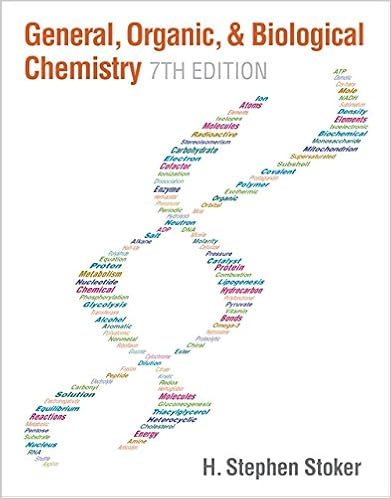# When solutions of strontium chloride and sodium

• Test Prep
• 81
• 91% (113) 103 out of 113 people found this document helpful

This preview shows page 38 - 44 out of 81 pages.

##### We have textbook solutions for you!
The document you are viewing contains questions related to this textbook.The document you are viewing contains questions related to this textbook.
Chapter 16 / Exercise 2
General, Organic, & Biological Chemistry
StokerExpert Verified
9. When solutions of strontium chloride and sodium carbonate react, which of the following are spectator ions? a) strontium ions and sodium ions b) sodium ions and chloride ions c) sodium ions and carbonate ions d) strontium ions and carbonate ions e) chloride ions and carbonate ions Finally, cancelled the repeated ions on the left and right side of the complete ionic equation to obtain the net ionic equation: Complete ionic equation: Sr+2 (aq) + 2Cl-(aq) + 2Na+(aq) + CO32-(aq) SrCO3(s) + 2Na+(aq) + 2ClSr+2 (aq) + 2Cl-(aq) + 2Na+(aq) + CO32-(aq) SrCO3(s) + 2Na+(aq) + 2ClNet ionic equation: Sr+2 (aq) + CO32-(aq) SrCO3Na+(aq) + 2Cl-are the spectator ions!
-(aq)
-(aq)
(s)
##### We have textbook solutions for you!
The document you are viewing contains questions related to this textbook.The document you are viewing contains questions related to this textbook.
Chapter 16 / Exercise 2
General, Organic, & Biological Chemistry
StokerExpert Verified
9. When solutions of strontium chloride and sodium carbonate react, which of the following are spectator ions?
-(aq)
-(aq)
(s)
10.With what volume of 5.00 MHF will 3.95 g of calcium hydroxide react completely, according to the following reaction?
10.With what volume of 5.00 MHF will 3.95 g of calcium hydroxide react completely, according to the following reaction?
2
10.With what volume of 5.00 MHF will 3.95 g of calcium hydroxide react completely, according to the following reaction? a)10.7 mL b) 107 mL c) 533 mL d) 21.3 mL e) 46.9 mL 2222HF + Ca(OH)CaF + 2HOThe first thing to calculate is how many moles of HF will redact with the given 3.95 g of Ca(OH)2: 3.95 g of Ca(OH)2 * 1 mol of Ca(OH)2* 2 mol of HF = 0.107 mol of HF 74.08 g of Ca(OH)2 1 mol of Ca(OH)
2
10.With what volume of 5.00 MHF will 3.95 g of calcium hydroxide react completely, according to the following reaction?
n
V
•••